Request your copy of SimphoSOFT® now

Webinars
Product brochureApplication notesIn many important practical situations, beam propagation through a nonlinear media can be accurately modeled by the following nonlinear Schrödinger equation (NLS)

 i Qη + Δ⊥Q + |Q|2 Q = 0 (NLS)

with a function Q0(ρ) as an incident radially symmetric pulse Q(ρ, η=0)= Q0(ρ) (here Qη = Q / η and the Laplacian Δ is given by  Δ= ∂2/∂ρ2 + (1/ρ)(∂/∂ρ),  η=z / Ldf  is a normalized propagation distance, and ρ= (x2+y2)1/2 / R0 is the normalized distance from the center of the pulse in the transverse two-dimensional plane, Ldf  is the diffraction length and R0 is the beam’s FW1/eM radius).

However, this model is prone to exponential growth or even singularity formation of a numerical solution in the focusing regions when self-focusing is a dominating process. Another model for nonlinear propagation, which proved to better handle self-focusing regimes , is a nonparaxial approximation (nopaxNLS) which, compared to NLS, has an extra term of the pulse second derivative

 i Qη + ē Qηη + Δ⊥Q + |Q|2 Q = 0 (nonpaxNLS)

with the following coefficient of nonparaxiality ē = (λ0 / 2πR0)2 for the incident pulse Q(ρ, η=0)= Q0(ρ) with the radius R0 and wavelength λ0. Both equations are derived from the following nonlinear Helmholtz (NHL) equation [7,8].

 Ezz(x,y,z,t) + Δ⊥E + (k0)2(1+(2n2/n0)|E|2) E = 0 (NHL)

by assuming no backscattering happens while pulse propagate the nonlinear sample. The model for such pulse can be represented by the following SVEA form with the complex conjugate term Q*(x,y,z,t), representing the backscattered part of the pulse, left out

 E(x,y,z,t) = R0 k0 (2n2/n0)1/2 Q(x,y,z,t) exp[ -i (ω0t– k0z) ]

If λ0 << R0 , which is the case in many situations, the nonparaxiality coefficient ē is negligibly small, so that nonparaxial approximation turns to NLS – paraxial approximation. NLS can be solved numerically as an example of an evolution problem (eta acts as the time variable in this case).

Problems with a numerical solution of paraxial NLS arise when the initial power of the incident pulse N0 = (||Q0||2)2=∫ |Q0(ρ)|2 ρ dρ exceeds a threshold power Nc = 1.86 [1,2], which happen in self-focusing regimes. What may happen in such cases is that the non-paraxial term ēQηη increases substantially to become as important as other terms in nonparaxial NLS, so that disregarding this term is no longer safe. Nonparaxial term plays as a balancing term, without which the Laplacian (diffraction) and the cubic nonlinearity (Kerr factor) become misbalanced in paraxial NLS, which leads to possible numerical collapses [3,4,5].

One solution would be to relax the nonparaxiality coefficient, introducing a linear damping ε which “turns on” the nonparaxial term near self-focusing regions only [6,7,8]. An extension of split-step method based on Padé approximation operators  allows solving nonparaxial NLS, where the nonparaxiality coefficient ε is adapted at each propagation step based on the behavior of the current solution.

 References  G. Fibich, A. Gaeta, Critical power for self-focusing in bulk media and in hollow waveguides, Opt. Lett. 25 (2000) 335–337.  M.I. Weinstein, Nonlinear Schrödinger equations and sharp interpolation estimate, Comm. Math. Phys. 87 (1983) 567–576.  N. Akhmediev, A. Ankiewicz, J.M. Soto-Crespo, Does the nonlinear Schrödinger equation correctly describe beam propagation?, Opt. Lett. 18 (1993) 411–413.  M.D. Feit, J.A. Fleck, Beam nonparaxiality, filament formation, and beam breakup in the self-focusing of optical beams, J. Opt. Soc. Amer. B Opt. Phys. 5 (1988) 633–640.  G. Fibich, Small beam nonparaxiality arrests self-focusing of optical beams, Phys. Rev. Lett. 76 (1996) 4356–4359.  G. Fibich, B. Ilan, S. Tsynkov, Backscattering and nonparaxiality arrest collapse of damped nonlinear waves, SIAM J. Appl. Math. 63 (5), (2003), 1718-1736.  G. Fibich A and S. Tsynkov B, Numerical solution of the nonlinear Helmholtz equation using nonorthogonal expansions, J. Comp. Phys. 210, (2005), 183-224.  G. Baruch, G. Fibich, and Semyon Tsynkov, Simulations of the nonlinear Helmholtz equation: arrest of beam collapse, nonparaxial solitons and counter-propagating beams, Opt. Express 16, 13323-13329 (2008).  K. Malakuti and E. Parilov, A split-step difference method for nonparaxial nonlinear Schrödinger equation at critical dimension, Appl. Num. Math. 61(7), 891-899 (2011).SimphoSOFT® propagation mathematical model is based on paraxaial approximation.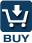SimphoSOFT® can be purchased as a single program and can be also configured with Energy Transfer add-on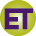, Multi-Beam add-on, Optimization add-on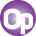, Z-scan add-on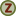, and MPA Info+ add-onfor an additional charge. Please, contact our sales staff for more information e-mail phone: +1 (973) 621-2340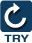You can request a remote evaluation of our software by filling out online form -- no questions asked to get it, no need to install it on your computer.